Create a new printableMath Worksheets

Sample - Click above to make a new math worksheet (PDF).
 Name _____________________________Date ___________________
Length and Weight
Circle the object with the longest side.
 1 * This is a pre-made sheet.Use the link at the top of the page for a printable page.
2.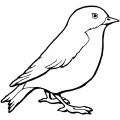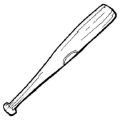3.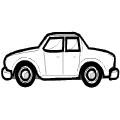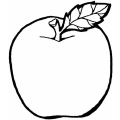4.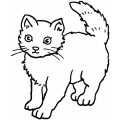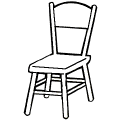Number the objects in order from shortest to longest. Compare using the longest side of each object.
Write 1 next to the object with the shortest length.  Write 3 next to the object with the longest length.

1.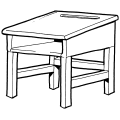______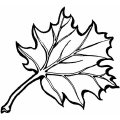______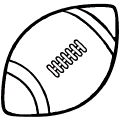______
2.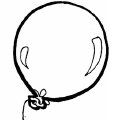______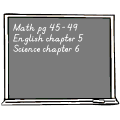______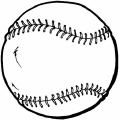______

Number the objects in order from longest to shortest. Compare using the longest side of each object.
Write 1 next to the object with the longest length.  Write 3 next to the object with the shortest length.

1.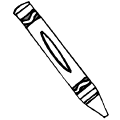____________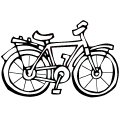______
2.__________________

 Key #2
 aKey #2
Estimate how long the object is. Circle the answer that gives the best estimate.
1.10 inches 10 feet
2.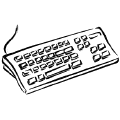1 inch 1 foot
3.11 inches 11 feet
4.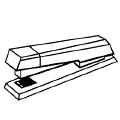6 inches 6 feet

Estimate how long the object is. Circle the answer that gives the best estimate.
1.2 meters 22 centimeters
2.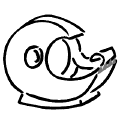10 centimeters 6 meters
3.100 centimeters 10 centimeters
4.25 centimeters 2 centimeters

Circle the object that is heavier.
1.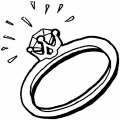2.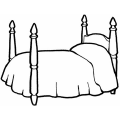3.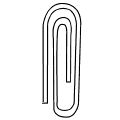4.Key #2
 aKey #2
Number the objects in order from lightest to heaviest.
Write 1 next to the lightest object.  Write 3 next to the heaviest object.

1.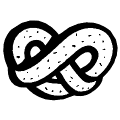__________________
2.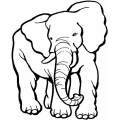______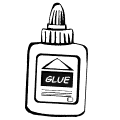____________

Estimate how much the object weighs. Circle the answer that gives the best estimate.
1.33 pounds 330 pounds
2.1 ounce 10 pounds
3.767 pounds 59 pounds
4.121 pounds 1 ounce

Estimate how much the object weighs. Circle the answer that gives the best estimate.
1.1,984 grams 2,048 kilograms
2.480 grams 48 kilograms
3.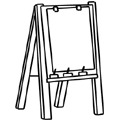17 kilograms 1 gram
4.3 kilograms 30 grams